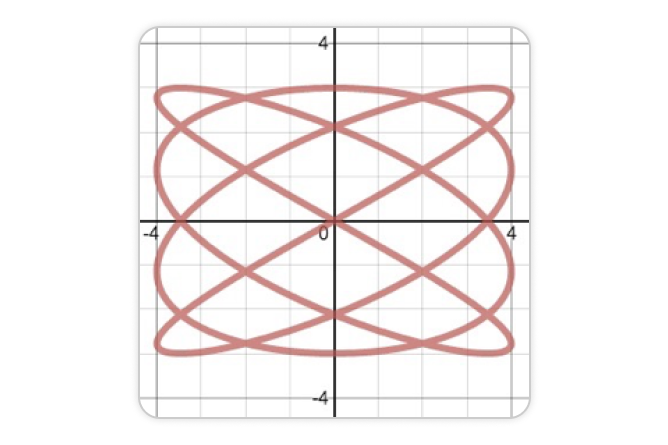# Parametric Equations

Graphing parametric equations on the Desmos Graphing Calculator is as easy as plotting an ordered pair. Instead of numerical coordinates, use expressions in terms of t, like (cos t, sin t). Graph lines, curves, and relations with ease.

Whether you’re interested in form, function, or both, you’ll love how Desmos handles parametric equations. Get started with the video on the right, then dive deeper with the resources and challenges below.

## Getting StartedTo plot a parametric curve, simply create an ordered pair where one or both coordinates are defined in terms of the parameter t.

By default, parametric curves are plotted for values of t in the interval [0, 1], but it is possible to adjust the domain manually using the inputs beneath the expression.It is also possible to fill a parametric curve by long-pressing the expression options menu and enabling the Fill option.Note that for the purpose of computing parametric fill, a curve’s interior is determined according to the non-zero winding rule.

## Parametric Equations in Action

### "The best way to learn is to do." – Paul Halmos### Graphing Challenges

Stretch your parametric skills with graphing challenges.